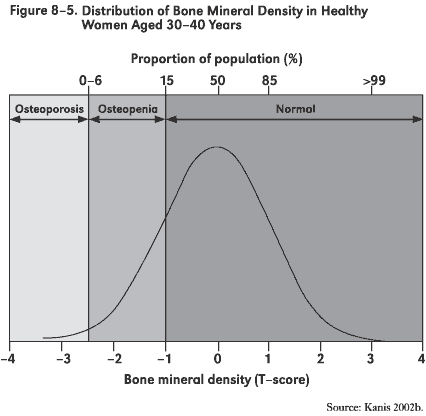## 2012-09-14 Class Notes

### Sampling Bias

Continued from Wednesday

### Library book sampling simulation

Continued from Wednesday

### Descriptions of Distributions

Main points

• Use the density itself if you are interested in the distribution.
• The smallest meaningful description of a distribution includes at least two attributes, which can be conveyed in two different but closely related ways:
• (1) center and (2) width
• (1) lower end of range to (2) upper end of range
• There is a conceptual trade-off between comprehensiveness and relevance.
• To have a comprehensive description, you would include the full range, for 100% of the distribution.
• But this would include even the outliers, members of the distribution that are not at all representive of the bulk of the distribution.
• To resolve the trade-off, it's typical to consider the central mass of the distribution. Two common ways:
1. The 50% coverage interval
2. The 95% coverage interval.
• For a rough and ready measure of the center and width of a distribution, we'll use the mean and standard deviation. These are not without difficulty (e.g., they are not robust to outliers) but they have very nice mathematical properties that simplify interpretation in complicated systems. It turns out that we can avoid the difficulties without sacrificing much in simplicity of interpretation by some simple strategies, e.g. the rank transform. We'll talk more about this when we have mastered interpretation in settings without problems relating to outliers.

### Example of Standard Deviation

Osteopenia.

Here's a quote from Wikipedia:

Osteopenia is a condition where bone mineral density is lower than normal. It is considered by many doctors to be a precursor to osteoporosis. However, not every person diagnosed with osteopenia will develop osteoporosis. More specifically, osteopenia is defined as a bone mineral density T-score between -1.0 and -2.5.

The article goes on:

The definition has been controversial. Steven R. Cummings, of the University of California, San Francisco, said in 2003 that “There is no basis, no biological, social, economic or treatment basis, no basis whatsoever” for using one standard deviation. Cummings added that “As a consequence, though, more than half of the population is told arbitrarily that they have a condition they need to worry about.” Quoted from this Gina Kolata articleBone density falls with age. The T-score is really just a Z-score, but compares a person to the distribution of young people. Some graphs

Osteopenia is defined so that about 1/6 of young people have it and much larger fractions of old people will have it.

### Means and Models

Important components of statistical models:

• The response variable. The quantity that varies from case to case whose spread is something we want to account for.

Example: wage varies from person to person.

• Explanatory variables. Other variables that we think may account for the response. For the present, we'll work with categorical variables. Each case falls into one group, and our model is that the groups are different from one another but the individuals within the group are all the same.

Example: wage might depend on sector of the economy or sex or something else.

``````cps = fetchData("CPS85")
``````
``````## Data CPS85 found in package.
``````

We can quantify the amount of variation using the standard deviation:

``````sd(wage, data = cps)
``````
``````##  5.139
``````
• The model values. For each group, there will be one model value. That is, according to our model, each case has a response value that is the value for that case's group.

Example: We might model wage by sex as “Men make \$3 per hour, women make \$5 per hour”

You'll never have to do this, but here's one way to implement that model:

``````fetchData("m155development.R")  # some new software
``````
``````## Retrieving from http://www.mosaic-web.org/go/datasets/m155development.R
``````
``````##  TRUE
``````
``````mymod = listFun(M = 3, F = 5)
``````

This is a function that takes “M” or “F” as an input and returns the model value.

Let's add these as variable `modvals` to the data

``````cps = transform(cps, modvals = mymod(sex))
``````
• The residuals. These tell how far the model values are from the actual values;
``````resids = with(data = cps, wage - modvals)
``````

One way to quantify the “goodness” of a model is with how far off the residuals are from their ideal value: zero. The mean square residual is a good measure of this:

``````mean(resids^2)
``````
``````##  55.53
``````

As with the arrows, there are two components to this: bias and variance.

``````mean(resids)  # bias, the systematic error
``````
``````##  5.106
``````
``````var(resids)  # the random error
``````
``````##  29.51
``````
``````sd(resids)  # the square root of the variance
``````
``````##  5.432
``````

The above model has a big bias. We want to choose the model values to make the sum of squared errors as small as possible. Ideally, we can make the bias zero. This is actually easy. We do it by fitting the model, adjusting the parameters to match the data as closely as possible. The `mm()` function will fit model values to the data.

• The fitted model values. Let's use as model values the means of the groups.
``````mean(wage ~ sex, data = cps)
``````
``````##     F     M
## 7.879 9.995
``````
``````f2 = listFun(M = 9.995, F = 7.879)
cps = transform(cps, means = f2(sex))
resids = with(cps, wage - means)
mean(resids^2)  # This model is better than the earlier one!
``````
``````##  25.25
``````
``````mean(resids)  # No bias!
``````
``````##  -0.0001124
``````
``````sd(resids)
``````
``````##  5.03
``````

The `mm()` function does these calculations for us in a convenient way, allowing us to extract the residuals and fitted model values without all the work.

``````mod = mm(wage ~ sex, data = cps)
mean(resid(mod)^2)
``````
``````##  25.25
``````
``````mean(resid(mod))  # No bias!
``````
``````##  -1.608e-16
``````
``````sd(resid(mod))
``````
``````##  5.03
``````

Any difference between the “by hand” version and the mm version is due to rounding off when typing in the mean values.
sd(resid)